# Check for Symmetrical Binary Tree

Problem Statement:

Check for Symmetrical Binary Trees

Write a program to check whether a binary tree is symmetrical or not.

Problem Description:

A symmetrical binary tree is a tree that forms a mirror of itself around the center. In other words, every node in the left subtree will have a mirror image in the right subtree.

Examples:

```Example 1: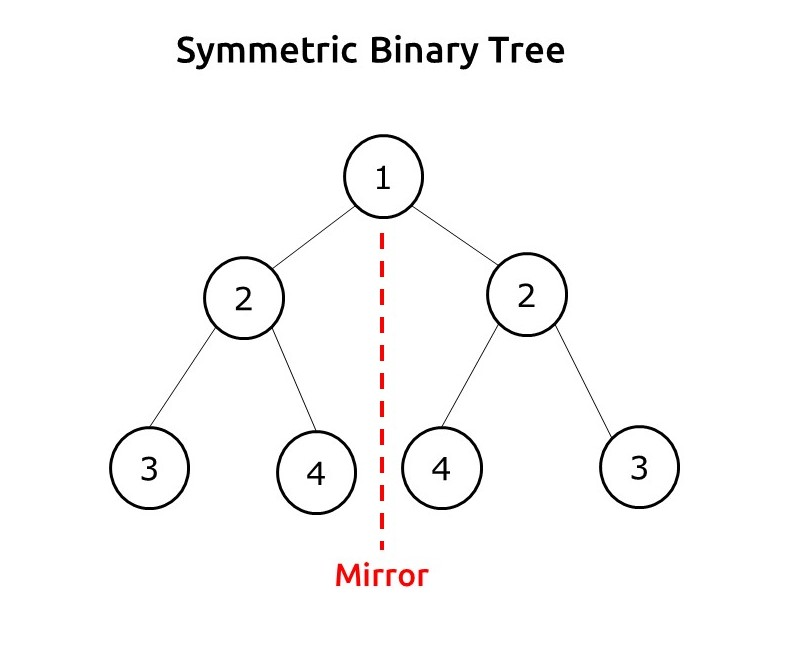```
```Example 2: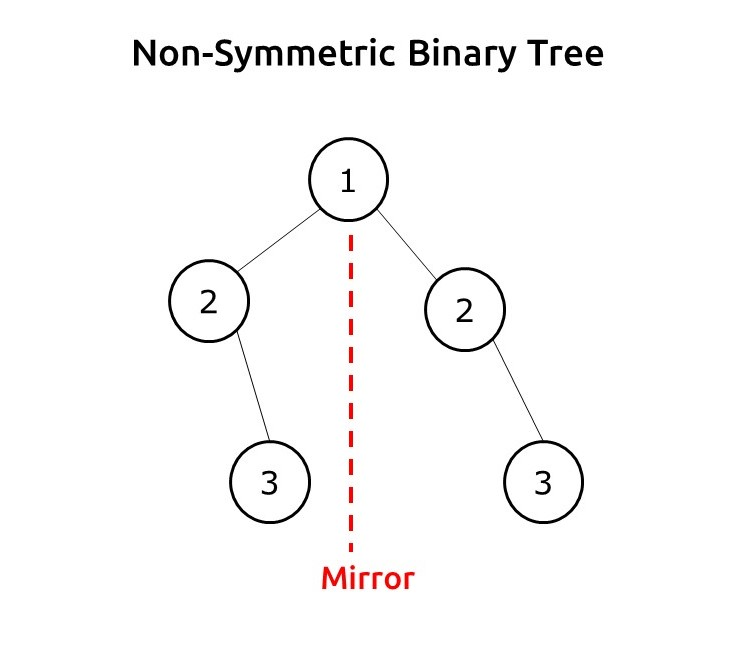```

Disclaimer: Don’t jump directly to the solution, try it out yourself first.

Pre-req: Tree Traversal

### Solution :

Intuition: We need to understand the property of the mirror. We can ignore the root node as it is lying on the mirror line. In the next level, for a symmetric tree, the node at the root’s left should be equal to the node at the root’s right.

If we take two variables root1 and root2 to represent the left child of root and right child of the root, then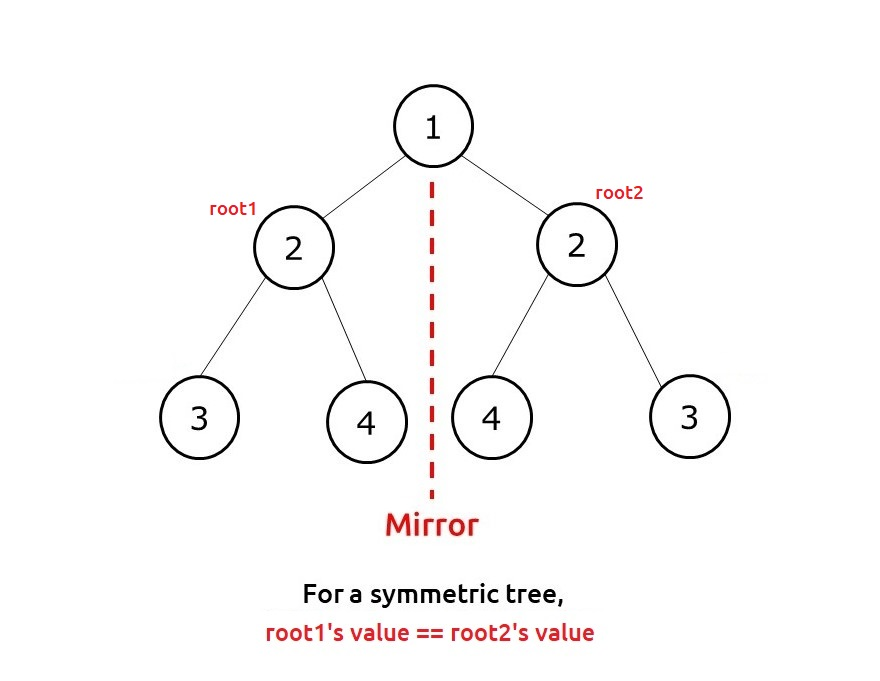Further, we need to understand that when root1’s value is equal to root2’s value, we need to further check for its children. As we are concerned about node positions through a mirror, root1’s left child should be checked with root2’s right child and root1’s right child should be checked with root2’s left child.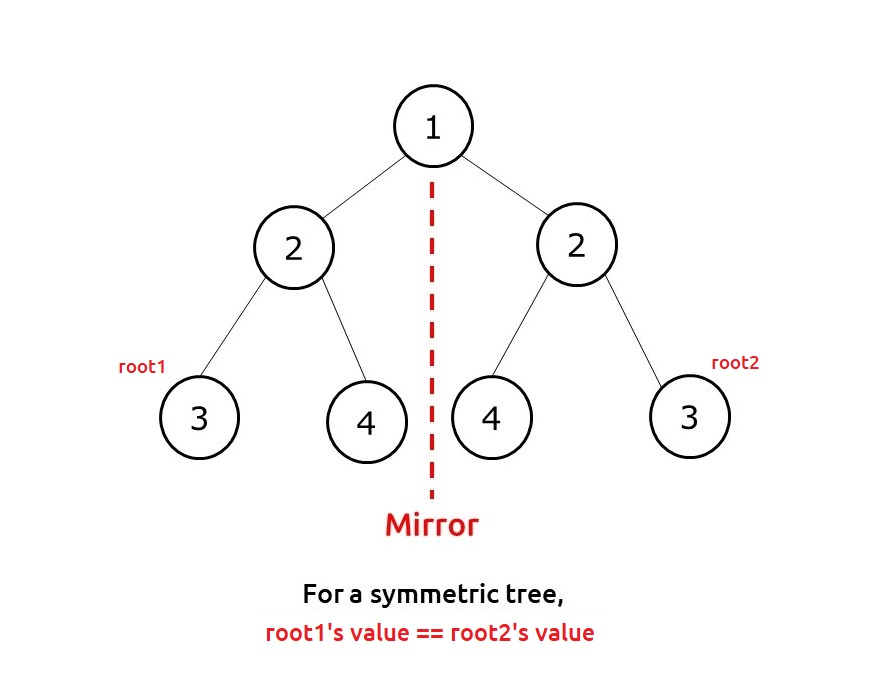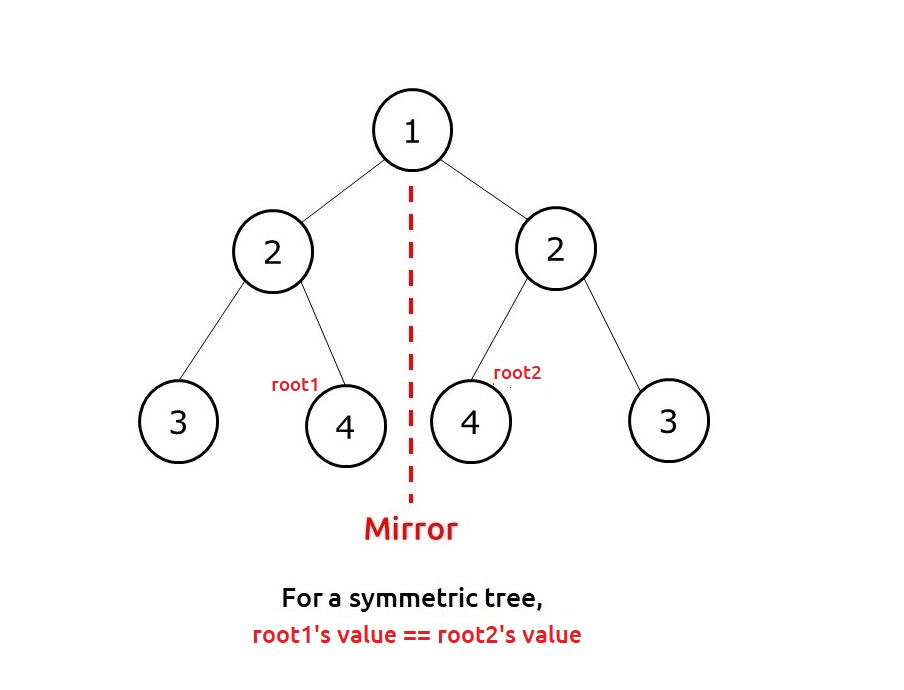Approach:

The algorithm steps can be summarized as follows::

• We take two variables root1 and root2 initially both pointing to the root.
• Then we use any tree traversal to traverse the nodes. We will simultaneously change root1 and root2 in this traversal function.
• For the base case, if both are pointing to NULL, we return true, whereas if only one points to NULL and other to a node, we return false.
• If both points to a node, we first compare their value, if it is the same we check for the lower levels of the tree.
• We recursively call the function to check the root1’s left child with root2’s right child; then we again recursively check the root1’s right child with root2’s left child.
• When all three conditions ( node values of left and right and two recursive calls) return true, we return true from our function else we return false.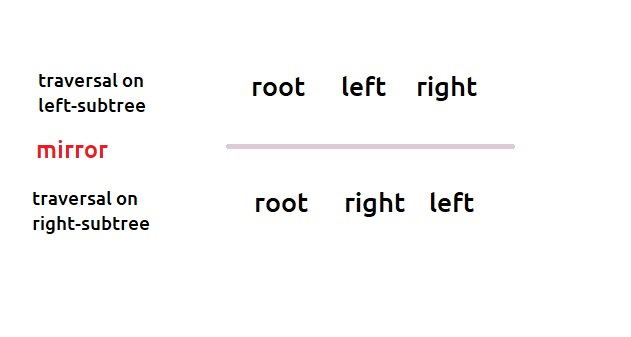Dry Run: In case you want to watch the dry run for this approach, please watch the video attached below.

Code:

## C++ Code

``````#include <bits/stdc++.h>

using namespace std;

struct node {
int data;
struct node * left, * right;
};

bool isSymmetricUtil(node * root1, node * root2) {
if (root1 == NULL || root2 == NULL)
return root1 == root2;
return (root1 -> data == root2 -> data) && isSymmetricUtil(root1 -> left, root2->
right) && isSymmetricUtil(root1 -> right, root2 -> left);
}
bool isSymmetric(node * root) {
if (!root) return true;
return isSymmetricUtil(root -> left, root -> right);
}

struct node * newNode(int data) {
struct node * node = (struct node * ) malloc(sizeof(struct node));
node -> data = data;
node -> left = NULL;
node -> right = NULL;

return (node);
}

int main() {

struct node * root = newNode(1);
root -> left = newNode(2);
root -> left -> left = newNode(3);
root -> left -> right = newNode(4);
root -> right = newNode(2);
root -> right -> left = newNode(4);
root -> right -> right = newNode(3);

bool res;
res = isSymmetric(root);

if (res)
cout << "The tree is symmetrical";
else cout << "The tree is NOT symmetrical";

return 0;
}

``````

Output:

The tree is symmetrical

Time Complexity: O(N)

Reason: We are doing simple tree traversal and changing both root1 and root2 simultaneously.

Space Complexity: O(N)

Reason: In the worst case (skewed tree), space complexity can be O(N).

## Java Code

``````import java.util.*;

class Node {
int data;
Node  left,  right;
Node(int data)
{
this.data=data;
left=null;
right=null;
}
}

class TUF{
static boolean isSymmetricUtil(Node  root1, Node  root2) {
if (root1 == null || root2 == null)
return root1 == root2;
return (root1 . data == root2 . data) && isSymmetricUtil(root1 . left, root2 .
right) && isSymmetricUtil(root1 . right, root2 . left);
}
static boolean isSymmetric(Node  root) {
if (root==null) return true;
return isSymmetricUtil(root . left, root . right);
}

public static void main(String args[]) {

Node  root = new Node(1);
root . left = new Node(2);
root . left . left = new Node(3);
root . left . right = new Node(4);
root . right = new Node(2);
root . right . left = new Node(4);
root . right . right = new Node(3);

boolean res;
res = isSymmetric(root);

if (res)
System.out.println("The tree is symmetrical");
else System.out.println("The tree is NOT symmetrical");

}
}

``````

Output:

The tree is symmetrical

Time Complexity: O(N)

Reason: We are doing simple tree traversal and changing both root1 and root2 simultaneously.

Space Complexity: O(N)

Reason: In the worst case (skewed tree), space complexity can be O(N).

Special thanks to Anshuman Sharma for contributing to this article on takeUforward. If you also wish to share your knowledge with the takeUforward fam, please check out this article